Get ICSE Solutions for Class 10 Mathematics Chapter 20 Probability for ICSE Board Examinations on ICSESolutions.com. We provide step by step Solutions for ICSE Mathematics Class 10 Solutions Pdf. You can download the Class 10 Maths ICSE Textbook Solutions with Free PDF download option.

## Probability Class 10 Maths ICSE Solutions

### Formulae

1. If all the outcomes of an experiment are equally likely and E is an event, then probability of event E, written by P (E), is given by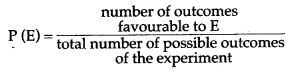2. 0 ≤ P (E) ≤ 1
3. P (not E) = 1 – P (E)
4. P (E) = 1 – P (not E)
5. P (E) + P (not E) = 1
6. The sum of the probabilities of all the elementary events of an experiment = 1
7. The probability of a sure event = 1
8. The probability of an impossible event = 0.

### Concept Based Questions

Question 1. An unbiased dice is thrown. What is the probability of getting a number other than 4.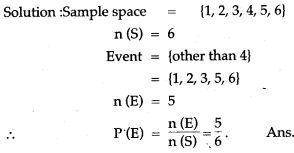Question 2. Two dice are thrown simulata- neously. Find the probability of getting six as the product.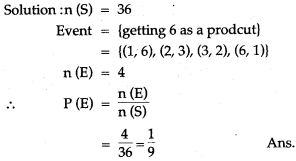Question 3. If the probability of winning a 5 game is 5/11. What is the probability of losing?Question 4. Find the probability of getting a tail in a throw of a coin.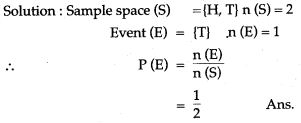Question 5. In a cricket match a batsman hits a boundary 6 times out of 30 balls he play’s. Find the probability that he did not hit the boundary?Question 6. It is known that a bax of 600 electric bulbs contain 12 defective bulbs. One bulb is taken out at random from this box. What is the probability that it is a non-defective bulb?Question 7. 1000 tickets of a lottery were sold and there are 5 prizes on these tickets. If Namita has purchased one lottery ticket, what is the probability of winning a prize?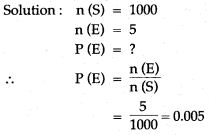Question 8. A coin is tossed 100 times with the following frequencies:
Head = 55, Tail = 45
find the probability for each event (i) head (ii) tail.Question 9. Namita tossed a coin once. What is the probability of getting (i) Head (ii) tail?Question 10. Two coins are tossed once. Find the probability of getting.Question 11. A die has 6 faces marked by the given numbers as shown below:
The die is thrown once. What is the probability of getting
(i) a positive integer.
(ii) an integer greater than – 3.
(iii) the smallest integer.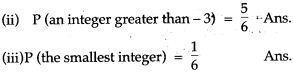Question 12. 1800 families with 2 children were selected randomly and the following data were recorded: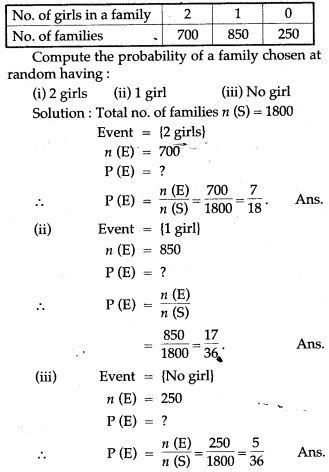Question 13. A card is drawn at random from a well shuffled pack of 52 cards. Find the probability that at the card drawn is neither a red card nor a qeen.Question 14. A dice is thrown once. What is the probability that the
(i) number is even
(ii) number is greater than 2?Question 15. A box contains some black balls and 30 white balls. If the probability of drawing a black ball is two-fifths of a white ball, find the number of black balls in the box.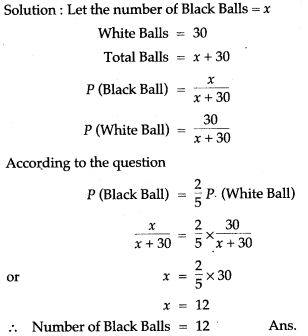Question 16. From a pack of 52 playing cards all cards whose numbers are multiples, of 3 are removed. A card is now drawn at random.
What is the probability that the card drawn is:
(i) a face card (King, Jack or Queen)
(ii) an even numbered red card?Question 17. One card is randomly drawn from a pack of 52 cards. Find the probability that:
(i) the drawn card is red.
(ii) the drawn card is an ace.
(iii) the drawn card is red and a king.
(iv) the drawn card is red or king.Question 18. One card is drawn from a pack of 52 cards, each of the 52 cards being equall likely to be drawn. Find the probability that the card drawn is (i) An ace, (ii) red, (iii) either red or king, (iv) red and a king, (v) a face card, (vi) a red face card, (vii) ‘2’ of spade, (viii) ’10’ of a blacksuit.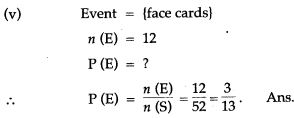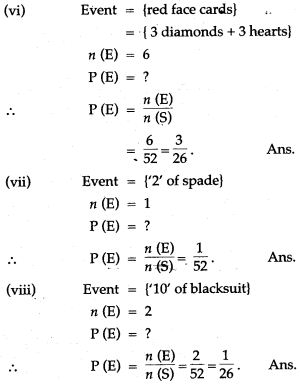Question 19. Two dice are thrown simulta-neously. Find the probability of getting:
(i) an even number as the stun, (ii) the sum as a prime number, (iii) a total of at least 10, (iv) a doublet of even number, (v) a multiple of 3 as the sum.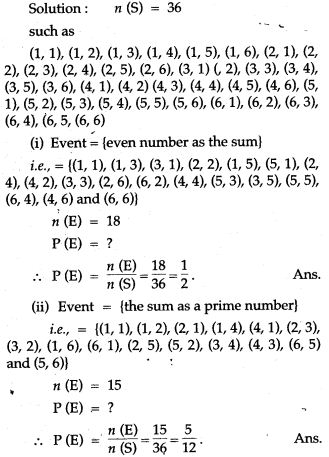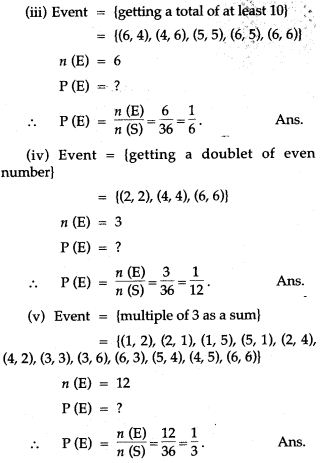Question 20. Find the probability that leap year selected at random, will contain 53 Sundays.